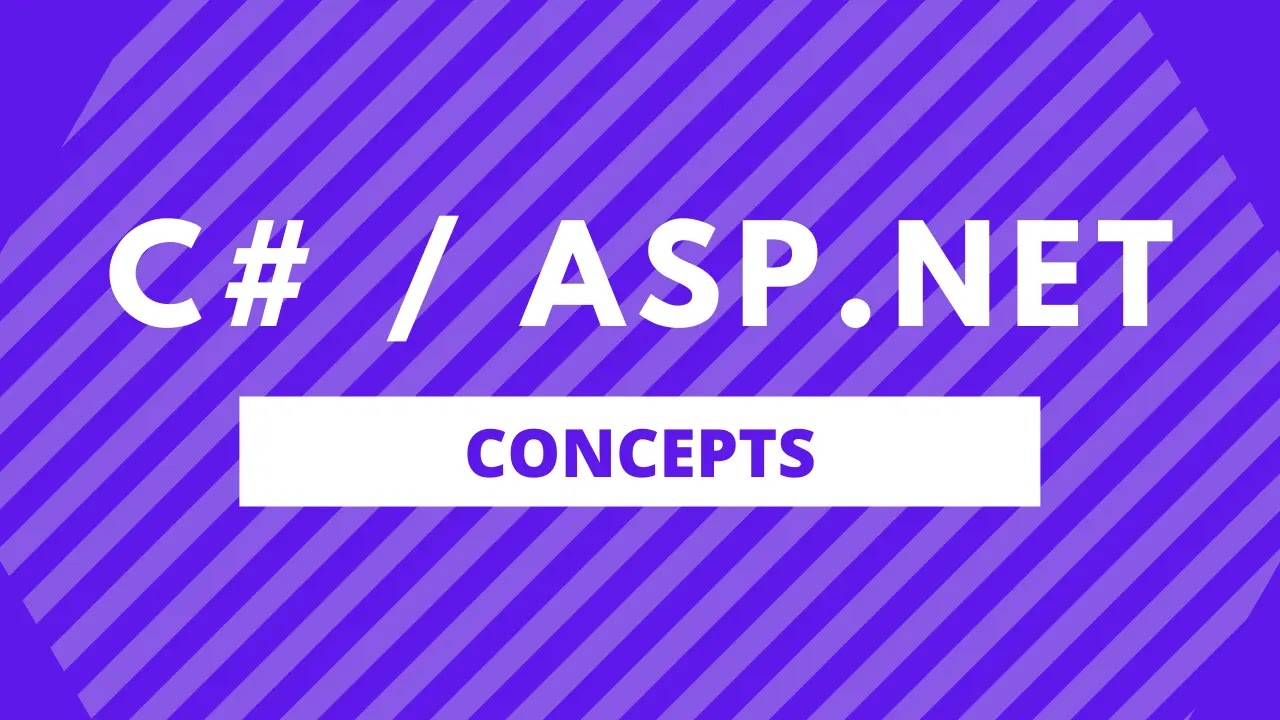# Convert Byte To String In C#

Sameer Saini March 06, 2023Converting a byte array to a string is a common task in C#, especially when working with data that has been encoded in bytes, such as image files or network packets. In this blog post, we'll explore several methods for converting byte arrays to strings in C#.

## Convert Byte To String Using Encoding.ASCII.GetString()

The Encoding.ASCII.GetString() method can be used to convert a byte array to a string using the ASCII character encoding. Here's an example:

``````byte[] bytes = new byte[] { 65, 66, 67 };
string result = Encoding.ASCII.GetString(bytes);``````

In this example, we create a byte array with the values 65, 66, and 67, which correspond to the ASCII characters 'A', 'B', and 'C'. We then use the Encoding.ASCII.GetString() method to convert the byte array to a string.

## Convert Byte To String Using Encoding.UTF8.GetString()

The Encoding.UTF8.GetString() method can be used to convert a byte array to a string using the UTF-8 character encoding, which supports a wider range of characters than ASCII. Here's an example:

``````byte[] bytes = new byte[] { 230, 152, 135, 229, 143, 139 };
string result = Encoding.UTF8.GetString(bytes);``````

In this example, we create a byte array with the values 230, 152, 135, 229, 143, and 139, which correspond to the UTF-8 encoded Chinese characters '你好'. We then use the Encoding.UTF8.GetString() method to convert the byte array to a string.

## Convert Byte To String Using BitConverter.ToString()

The BitConverter.ToString() method can be used to convert a byte array to a string of hexadecimal digits. Here's an example:

``````byte[] bytes = new byte[] { 0x12, 0x34, 0x56 };
string result = BitConverter.ToString(bytes).Replace("-", "");``````

In this example, we create a byte array with the values 0x12, 0x34, and 0x56. We then use the BitConverter.ToString() method to convert the byte array to a string of hexadecimal digits, and remove the '-' separator between each pair of digits.

## Conclusion

In this blog post, we've explored several methods for converting byte arrays to strings in C#. Depending on the specific requirements of your application, one of these methods may be more suitable than the others. By understanding the capabilities and limitations of each method, you can choose the one that best meets your needs.